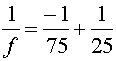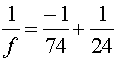Examples for optical instruments

Example #1

Problem:

a.) Grandpa's far point is 75 cm. What is the prescription (refractive power in diopters) for Grandpa's contact lenses?

Solution:

A diverging lens will make an image of a far away object at its focal length. Therefore, the focal length is negative 75 cm, and the prescription is

-1.33 diopters

b.) What is his prescription (in diopters) for eye glasses?

Solution:

The image must be brought to 74 cm. Therefore the prescription is

-1.35 diopters

c.) Grandma's near point is 75 cm. What is the prescription (in diopters) for Grandpa's contact lenses?

Solution:

Choose the focal length such that an object at the normal near point of 25 cm produces an image where Grandma can see it, at 75 cm-> f = 37.5 cm, and the prescription is

2.33 diopters

d.) What is her prescription for eye glasses?

Solution:

The same as above, only the normal near point is 24 cm from the glasses and her near point is 74 cm from the lenses,-> f = 35.5 m.

2.81 diopters

Example #2

Problem:

a.) A simple two-lens telescope's eyepiece has a focal length of 10 cm. If one wishes the magnification of the telescope to by a factor of 40, what is the length of the telescope?

Solution:

The second lens must have a focal length 40 times longer, 400 cm. The overall length is thus

4.10 m

Optical instrument's index